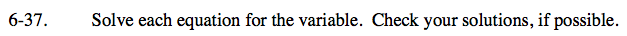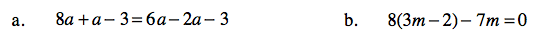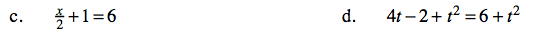### Home > CAAC > Chapter 6 > Lesson 6.2.1 > Problem6-37

6-37.
1. Solve each equation for the variable. Check your solutions, if possible. Homework Help ✎

1. 8a + a − 3 = 6a − 2a − 3

2. 8(3m − 2) − 7m = 0

3.+ 1 = 6

4.4t − 2t2 = 6t 2Combine like terms.

9a − 3 = 4a − 3

Get the variable on a side by itself.

5a = 0

a = 0

Follow the steps in part (a).

$m=\frac{16}{17}$Follow the steps in part (a).

Get x on a side by itself and then multiply both sides by 2.

Follow the steps in part (a).